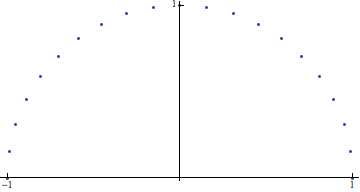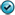Functions

In mathematics, functions are the rules that when applied, take one thing and produce another. They often involve numerical quantities, but this is not required.

To make this a bit more concrete -- each of the following gives an example of applying a function:

• Squaring $-5$ to get $25$;
• Calculating the value of $x^2+x - 7$, when $x=6$ and getting $35$;
• Finding the letter two letters after "P" is "R";
• Looking up the year the book Battlefield Earth by L. Ron Hubbard was first published and finding it was in 1982.

In each case, there is a input element and some associated output element:

InputOutput
$-5$$25 6$$35$
the letter "P"The letter "R"
the book Battlefield Earththe year $1982$

We can think of a function as the rule that gives us an output element for some allowed input element. This rule could be as simple as "square the input", or "look up the year the input was first published", or it might be much, much more complicated.

Of course, for a given function -- we likely won't be able to apply it's "rule" to just any input element we might choose. There will frequently be many elements that simply don't make sense as inputs for a given function.

For example, it makes sense to find the first-published date for Battlefield Earth by L. Ron Hubbard, Dracula by Bram Stoker, and A Wrinkle in Time by Madeleine L'Engle, but we can't find the first-published date for the value $-5$. Similarly, we can square the real values $-5$ and $6$, but attempting to square the letter "P" or the book A Wrinkle in Time clearly results in a problem.

Other times, even though we might be able to find the output of a function for a given input, we may still wish to disallow the application of the function to that input.

For example, physics tells us that if we throw a ball vertically into the air with a velocity of $40$ ft/s, its height (in feet) after $t$ seconds will be given by the value of $-16t^2 + 40t$. The table below shows us some heights attained at various times $t$. $$\begin{array}{c|c} t \textrm{ seconds} & \textrm{height in feet}\\ 1 & 24\\\hline 1.25 & 25\\\hline 1.5 & 24\\\hline 2 & 16\\ \end{array}$$ We can certainly calculate the value of this polynomial when $t=-2$ as well (it's $-112$), but that value can't be the height of the ball, as the ball hasn't even been thrown yet! Since something similar happens every time $t$ is negative, we allow only non-negative real values as inputs to this function.

So for a given function, there is a set of elements that we allow to be used as inputs for that function, and other elements that we don't.

Output elements of a particular function often share some common characteristic as well. For example, they might all be real values, or letters, or years, etc... However, not every element that has the common characteristic need be an output element.

For example, just because the first-published dates in our earlier example were all years does not mean that every year is an output for some book. Some years might not make sense, like 1237, a year well before the invention of the printing press. Similarly, every square of a real value is itself a real value. However, the set of all outputs of the "squaring function" is not the set of all real values. If it were, what real value squared would produce $-1$?

The best we can say is that these common characteristics helps us identify a reasonable set of potential outputs for the function in question.

Putting all this together, we can say that for any given function, there is some set of allowed inputs and some set of potential outputs that make sense with the function.

Let's give these two sets some names and formally define what we mean by a function:

A function $f$ is a rule that assigns to each element $x$ of some set (of inputs) called the domain of $f$ exactly one element in some other set (of potential outputs) called the co-domain of $f$. We denote the element of the co-domain associated with $x$ by $f(x)$, reading the same as either "the value of $f$ at $x$" or more briefly, just "$f$ of $x$".

Describing Functions

Describing a function is often done in one of four ways:

• words (i.e., a verbal description),
• a table,
• a graph, or
• an explicit formula.
We have already seen the first way above when we used words like "square the input", or "find the year the input book was first published", etc. This method of describing functions is often the most easily understood, but it is frequently the least useful for any further analysis and/or manipulation, without "translating" it into one of the other representations first.

When there are a small number of inputs, perhaps the simplest way to describe a function consists of just making a table listing the elements of its domain and the corresponding elements of its co-domain each has been assigned.

As an example, consider the function with domain $\{1,2,3,4\}$ and co-domain $\{4,5,6,7\}$ whose "rule" is given by the following: $$\begin{array}{|c|c|} \hline x & f(x)\\\hline 1 & 4\\\hline 2 & 6\\\hline 3 & 7\\\hline 4 & 4\\\hline \end{array}$$

As the above demonstrates, one should remember that the definition for a function given above does not require that all of the elements of the co-domain end up associated with some element of the domain. As such, we define the range of a function to be the elements of it's co-domain that are actually assigned to elements of it's domain.††

When there are many inputs, a table representation can be unwieldy, and unintentionally obscure important properties of the function. For example, do you notice anything interesting about the following function?

$$\begin{array}{|c|c|}\hline x & f(x)\\\hline -1 & 0 \\\hline -0.987688 & 0.156434 \\\hline -0.951057 & 0.309017 \\\hline -0.891007 & 0.45399 \\\hline -0.809017 & 0.587785 \\\hline -0.707107 & 0.707107 \\\hline -0.587785 & 0.809017 \\\hline -0.45399 & 0.891007 \\\hline -0.309017 & 0.951057 \\\hline -0.156434 & 0.987688 \\\hline 0 & 1 \\\hline 0.156434 & 0.987688 \\\hline 0.309017 & 0.951057 \\\hline 0.45399 & 0.891007 \\\hline 0.587785 & 0.809017 \\\hline 0.707107 & 0.707107 \\\hline 0.809017 & 0.587785 \\\hline 0.891007 & 0.45399 \\\hline 0.951057 & 0.309017 \\\hline 0.987688 & 0.156434 \\\hline 1 & 0 \\\hline \end{array}$$

Look what happens when we treat each input-output pair as coordinates and plot the associated points, as shown below. Clearly from the picture that results, this function's rule is somehow related to points spaced evenly apart on a semicircle of radius $1$ and with center at the origin.When all of the input and output elements for a function are numerical values, treating the input-output pairs as coordinates and plotting the associated points often reveals things impossible to see in a function's table form. As such, we add this technique to our toolbelts to deal with functions whose domain and co-domain consist of real values, calling the plotted points the graph of the function in question.

Graphs do have their downsides, however. For one, they are inherently approximate in nature. For example, if only the graph of $f$ above was given to you, without the corresponding table -- could you find to thirty decimal places the value of $f(0.951057)$? Probably not.

There is another downside that is shared by both graphs and tables. What happens when the domain is infinite? Certainly, creating a table with an infinite number of rows is impossible -- and without a complete table of input-output pairs, or other information about the nature of the function, how do we know what the entire graph looks like?

This problem brings us to yet another way to represent a function -- by an explicit formula. Not every function can be represented in this way, but most of the ones in which we will be interested can. An explicit formula gives us a way to calculate the output of the function for any given input, as seen in the examples below.

Examples
1. Suppose $f(x) = x^3$ for all real values $x$, and then find $f(2)$,  $f(-3)$,  $f(a)$,  $f(b+h)$,  and  $f(x+h)$.2. Suppose $g(x) = x^2 + x + h$ for all real values $x$, and then find $f(3)$,  $f(h)$,  $f(a+b)$,  and  $f(x+h)$.Implicit Domains

When we are given a function described by a formula, but where the domain has not been explicitly identified, we generally assume its domain to be the set of all real numbers††† that can be used as input (i.e., the set of real numbers that don't cause any problems when used as input -- such as making the output undefined or non-real). We frequently refer to this set as the implicit domain.

For example, suppose $$f(x) = \frac{x^2 + 1}{x-7} + \sqrt{10-x^2}$$ To find the aforementioned implicit domain for this function, we note that the only things that could keep this function from producing some real-valued output would be if we divided by zero in the first term, or if we took the square root of a negative value in the second term. The implicit domain should then consist of all real numbers $x$ that don't create one of those situations.

The denominator of the first term of $f(x)$ is zero only if $x=7$, while the expression under the square root in the second term is only negative if $10-x^2 \lt 0$. Solving the inequality tells us the second term is non-real when $x \gt \sqrt{10}$ or $x \lt -\sqrt{10}$.

Remember, these are the "problem values" of $x$. The implicit domain is the set of all of the other "non-problem values" of $x$. So throwing out $7$ and everything farther than $\sqrt{10}$ from zero on the real number line -- we are left with an implicit domain consisting of the union of two intervals, $$[-\sqrt{10},7) \cup (7,\sqrt{10}]$$

The Vertical Line Test

Recall that the definition of a function detailed above required any input value $x$ in the domain be associated with exactly one output value $y$. Think about what this means graphically for a function $f(x)$, whose domain and range are subsets of the real numbers. For any real number $x_0$, either this value is not in domain of $f$ and there is no point on the vertical line $y=x_0$, or this value is in the domain of $f$ and there is a single point on the vertical line $y=x_0$, namely $(x_0,f(x_0))$.

So vertical lines drawn on the graph of a function intersect the function at most once.

Thus, if we are looking at a graph and can spot a vertical line that intersects the graph more than once, we immediately know that the graph does not represent a function.

When we check to see if a graph can represent a function in this way, we are said to be applying the vertical line test.

†:In truth, we might even be more restrictive than that, depending on the time at which the ball strikes the ground. We certainly wouldn't want to consider any $t$ values past that time either...

††: The use of the word "range" can be somewhat ambiguous as some authors use it to describe the co-domain instead. To avoid this ambiguity, the "image of $f$" should really be used in it's place. Although for now, in order to stay closer to students' probable prior experience with such things, we will continue to use the word "range".

†††: This is not entirely true. What we take to be the implicit domain depends a lot on the context for the function. It might be that our default choice in some situations is the set of complex values of the form $a + bi$, or the set of $n$-dimensional vectors, or something else entirely. But for the purposes of most of the functions you will deal with in precalculus, let us assume that our inputs and outputs are generally real numbers.